# Mean State

Period Mean (original grids) [K]
Model Period Mean (intersection) [K]
Model Period Mean (complement) [K]
Benchmark Period Mean (intersection) [K]
Benchmark Period Mean (complement) [K]
Bias [K]
RMSE [K]
Phase Shift [months]
Bias Score 
RMSE Score 
Seasonal Cycle Score 
Spatial Distribution Score 
Interannual Variability Score 
Overall Score 
Benchmark [-] 299.
CRUNCEPv7 [-] 299. 299. 301. 298. 300. 0.167 0.744 0.331 0.623 0.791 0.965 0.983 0.958 0.852
GSWP3v1 [-] 299. 299. 300. 298. 300. 0.273 0.683 0.342 0.666 0.785 0.969 1.00 0.957 0.860
WFDEI [-] 299. 299. 301. 298. 300. 0.327 1.03 0.426 0.614 0.522 0.961 0.989 0.881 0.748
Period Mean (original grids) [K]
Model Period Mean (intersection) [K]
Model Period Mean (complement) [K]
Benchmark Period Mean (intersection) [K]
Benchmark Period Mean (complement) [K]
Bias [K]
RMSE [K]
Phase Shift [months]
Bias Score 
RMSE Score 
Seasonal Cycle Score 
Spatial Distribution Score 
Interannual Variability Score 
Overall Score 
Benchmark [-] 300.
CRUNCEPv7 [-] 301. 301. 301. 300. 300. 0.445 0.818 0.178 0.746 0.849 0.986 0.997 0.968 0.899
GSWP3v1 [-] 301. 301. 301. 300. 300. 0.412 0.773 0.166 0.763 0.856 0.987 0.989 0.962 0.902
WFDEI [-] 300. 300. 301. 300. 300. 0.266 1.33 0.258 0.771 0.623 0.981 0.996 0.908 0.817
Period Mean (original grids) [K]
Model Period Mean (intersection) [K]
Model Period Mean (complement) [K]
Benchmark Period Mean (intersection) [K]
Benchmark Period Mean (complement) [K]
Bias [K]
RMSE [K]
Phase Shift [months]
Bias Score 
RMSE Score 
Seasonal Cycle Score 
Spatial Distribution Score 
Interannual Variability Score 
Overall Score 
Benchmark [-] 295.
CRUNCEPv7 [-] 296. 296. 297. 295. 297. 0.455 0.854 0.360 0.713 0.862 0.955 1.00 0.946 0.890
GSWP3v1 [-] 296. 296. 296. 295. 297. 0.471 0.825 0.298 0.722 0.866 0.964 0.998 0.959 0.896
WFDEI [-] 295. 295. 297. 295. 297. 0.295 1.14 0.480 0.734 0.685 0.941 1.00 0.880 0.821
Period Mean (original grids) [K]
Model Period Mean (intersection) [K]
Model Period Mean (complement) [K]
Benchmark Period Mean (intersection) [K]
Benchmark Period Mean (complement) [K]
Bias [K]
RMSE [K]
Phase Shift [months]
Bias Score 
RMSE Score 
Seasonal Cycle Score 
Spatial Distribution Score 
Interannual Variability Score 
Overall Score 
Benchmark [-] 262.
CRUNCEPv7 [-] 260. 260. 263. 262. 266. -1.03 1.64 0.0159 0.920 0.941 0.999 0.999 0.919 0.953
GSWP3v1 [-] 262. 261. 267. 262. 266. 0.243 1.14 0.0153 0.941 0.956 0.999 1.00 0.938 0.965
WFDEI [-] 260. 260. 263. 262. 266. -1.58 3.38 0.0269 0.895 0.832 0.998 1.00 0.917 0.913
Period Mean (original grids) [K]
Model Period Mean (intersection) [K]
Model Period Mean (complement) [K]
Benchmark Period Mean (intersection) [K]
Benchmark Period Mean (complement) [K]
Bias [K]
RMSE [K]
Phase Shift [months]
Bias Score 
RMSE Score 
Seasonal Cycle Score 
Spatial Distribution Score 
Interannual Variability Score 
Overall Score 
Benchmark [-] 299.
CRUNCEPv7 [-] 299. 299. 300. 299. 299. -0.00747 0.779 0.604 0.420 0.686 0.915 0.999 0.883 0.765
GSWP3v1 [-] 299. 299. 300. 299. 299. 0.194 0.749 0.487 0.429 0.717 0.930 0.957 0.908 0.776
WFDEI [-] 299. 299. 300. 299. 299. 0.338 0.948 0.886 0.385 0.411 0.862 0.994 0.865 0.655
Period Mean (original grids) [K]
Model Period Mean (intersection) [K]
Model Period Mean (complement) [K]
Benchmark Period Mean (intersection) [K]
Benchmark Period Mean (complement) [K]
Bias [K]
RMSE [K]
Phase Shift [months]
Bias Score 
RMSE Score 
Seasonal Cycle Score 
Spatial Distribution Score 
Interannual Variability Score 
Overall Score 
Benchmark [-] 271.
CRUNCEPv7 [-] 271. 270. 274. 271. 274. -0.709 1.06 0.00815 0.940 0.959 0.999 0.999 0.981 0.973
GSWP3v1 [-] 271. 271. 275. 271. 274. -0.00239 0.633 0.00842 0.967 0.971 0.999 1.00 0.979 0.981
WFDEI [-] 270. 270. 273. 271. 274. -1.29 3.32 0.00841 0.905 0.805 0.999 0.999 0.922 0.906
Period Mean (original grids) [K]
Model Period Mean (intersection) [K]
Model Period Mean (complement) [K]
Benchmark Period Mean (intersection) [K]
Benchmark Period Mean (complement) [K]
Bias [K]
RMSE [K]
Phase Shift [months]
Bias Score 
RMSE Score 
Seasonal Cycle Score 
Spatial Distribution Score 
Interannual Variability Score 
Overall Score 
Benchmark [-] 280.
CRUNCEPv7 [-] 280. 280. 287. 280. 285. 0.0112 1.17 0.0268 0.900 0.949 0.998 1.00 0.963 0.960
GSWP3v1 [-] 281. 280. 288. 280. 285. 0.317 1.10 0.0205 0.899 0.957 0.999 1.00 0.966 0.963
WFDEI [-] 280. 280. 287. 280. 285. -0.284 2.48 0.0400 0.891 0.820 0.997 1.00 0.908 0.906
Period Mean (original grids) [K]
Model Period Mean (intersection) [K]
Model Period Mean (complement) [K]
Benchmark Period Mean (intersection) [K]
Benchmark Period Mean (complement) [K]
Bias [K]
RMSE [K]
Phase Shift [months]
Bias Score 
RMSE Score 
Seasonal Cycle Score 
Spatial Distribution Score 
Interannual Variability Score 
Overall Score 
Benchmark [-] 297.
CRUNCEPv7 [-] 297. 297. 300. 297. 300. 0.186 1.14 0.178 0.745 0.881 0.982 0.998 0.939 0.905
GSWP3v1 [-] 297. 297. 300. 297. 300. 0.0966 1.09 0.168 0.767 0.871 0.983 1.00 0.946 0.906
WFDEI [-] 297. 297. 300. 297. 300. 0.140 1.54 0.201 0.759 0.726 0.981 0.999 0.916 0.851
Period Mean (original grids) [K]
Model Period Mean (intersection) [K]
Model Period Mean (complement) [K]
Benchmark Period Mean (intersection) [K]
Benchmark Period Mean (complement) [K]
Bias [K]
RMSE [K]
Phase Shift [months]
Bias Score 
RMSE Score 
Seasonal Cycle Score 
Spatial Distribution Score 
Interannual Variability Score 
Overall Score 
Benchmark [-] 264.
CRUNCEPv7 [-] 263. 263. 264. 264. 264. -1.17 1.53 0.0147 0.924 0.942 0.999 0.999 0.969 0.963
GSWP3v1 [-] 264. 264. 267. 264. 264. 0.0228 0.741 0.0121 0.967 0.964 0.999 1.00 0.961 0.976
WFDEI [-] 262. 262. 263. 264. 264. -1.68 4.03 0.0104 0.894 0.784 0.999 0.999 0.956 0.903
Period Mean (original grids) [K]
Model Period Mean (intersection) [K]
Model Period Mean (complement) [K]
Benchmark Period Mean (intersection) [K]
Benchmark Period Mean (complement) [K]
Bias [K]
RMSE [K]
Phase Shift [months]
Bias Score 
RMSE Score 
Seasonal Cycle Score 
Spatial Distribution Score 
Interannual Variability Score 
Overall Score 
Benchmark [-] 295.
CRUNCEPv7 [-] 295. 295. 293. 295. 294. 0.337 0.625 0.0795 0.888 0.931 0.994 0.999 0.954 0.950
GSWP3v1 [-] 295. 295. 294. 295. 294. 0.502 0.712 0.0784 0.873 0.929 0.995 1.00 0.966 0.949
WFDEI [-] 295. 295. 293. 295. 294. 0.415 1.49 0.150 0.871 0.756 0.990 0.998 0.932 0.884
Period Mean (original grids) [K]
Model Period Mean (intersection) [K]
Model Period Mean (complement) [K]
Benchmark Period Mean (intersection) [K]
Benchmark Period Mean (complement) [K]
Bias [K]
RMSE [K]
Phase Shift [months]
Bias Score 
RMSE Score 
Seasonal Cycle Score 
Spatial Distribution Score 
Interannual Variability Score 
Overall Score 
Benchmark [-] 282.
CRUNCEPv7 [-] 282. 282. 284. 282. 286. -0.0854 0.985 0.106 0.894 0.923 0.993 0.996 0.945 0.946
GSWP3v1 [-] 282. 282. 284. 282. 286. 0.167 0.867 0.113 0.898 0.937 0.992 1.00 0.970 0.956
WFDEI [-] 281. 281. 283. 282. 286. -0.875 2.55 0.211 0.840 0.734 0.986 0.988 0.956 0.873
Period Mean (original grids) [K]
Model Period Mean (intersection) [K]
Model Period Mean (complement) [K]
Benchmark Period Mean (intersection) [K]
Benchmark Period Mean (complement) [K]
Bias [K]
RMSE [K]
Phase Shift [months]
Bias Score 
RMSE Score 
Seasonal Cycle Score 
Spatial Distribution Score 
Interannual Variability Score 
Overall Score 
Benchmark [-] 287.
CRUNCEPv7 [-] 282. 286. 244. 287. 292. -0.0764 1.03 0.150 0.821 0.899 0.984 0.998 0.952 0.925
GSWP3v1 [-] 283. 287. 249. 287. 292. 0.241 0.901 0.136 0.831 0.906 0.986 1.00 0.959 0.931
WFDEI [-] 282. 286. 247. 287. 292. -0.298 2.09 0.209 0.814 0.719 0.977 0.998 0.914 0.857
Period Mean (original grids) [K]
Model Period Mean (intersection) [K]
Model Period Mean (complement) [K]
Benchmark Period Mean (intersection) [K]
Benchmark Period Mean (complement) [K]
Bias [K]
RMSE [K]
Phase Shift [months]
Bias Score 
RMSE Score 
Seasonal Cycle Score 
Spatial Distribution Score 
Interannual Variability Score 
Overall Score 
Benchmark [-] 296.
CRUNCEPv7 [-] 296. 296. 297. 296. 297. 0.266 0.870 0.130 0.890 0.940 0.990 1.00 0.964 0.954
GSWP3v1 [-] 296. 296. 296. 296. 297. 0.213 0.830 0.134 0.897 0.939 0.990 0.999 0.963 0.955
WFDEI [-] 296. 296. 297. 296. 297. -0.179 1.70 0.193 0.887 0.802 0.985 0.999 0.908 0.897
Period Mean (original grids) [K]
Model Period Mean (intersection) [K]
Model Period Mean (complement) [K]
Benchmark Period Mean (intersection) [K]
Benchmark Period Mean (complement) [K]
Bias [K]
RMSE [K]
Phase Shift [months]
Bias Score 
RMSE Score 
Seasonal Cycle Score 
Spatial Distribution Score 
Interannual Variability Score 
Overall Score 
Benchmark [-] 265.
CRUNCEPv7 [-] 264. 264. 261. 265. 266. -0.968 1.47 0.0112 0.921 0.930 0.999 0.997 0.943 0.953
GSWP3v1 [-] 265. 265. 266. 265. 266. 0.264 0.995 0.0182 0.944 0.950 0.999 1.00 0.958 0.967
WFDEI [-] 264. 264. 261. 265. 266. -1.37 3.46 0.0347 0.896 0.786 0.998 0.994 0.924 0.897
Period Mean (original grids) [K]
Model Period Mean (intersection) [K]
Model Period Mean (complement) [K]
Benchmark Period Mean (intersection) [K]
Benchmark Period Mean (complement) [K]
Bias [K]
RMSE [K]
Phase Shift [months]
Bias Score 
RMSE Score 
Seasonal Cycle Score 
Spatial Distribution Score 
Interannual Variability Score 
Overall Score 
Benchmark [-] 296.
CRUNCEPv7 [-] 295. 295. 298. 296. 298. 0.180 1.21 0.275 0.669 0.842 0.977 1.00 0.925 0.876
GSWP3v1 [-] 296. 295. 298. 296. 298. 0.383 1.19 0.243 0.669 0.860 0.978 0.997 0.945 0.885
WFDEI [-] 295. 295. 298. 296. 298. 0.174 1.62 0.259 0.678 0.672 0.981 0.999 0.911 0.819
Period Mean (original grids) [K]
Model Period Mean (intersection) [K]
Model Period Mean (complement) [K]
Benchmark Period Mean (intersection) [K]
Benchmark Period Mean (complement) [K]
Bias [K]
RMSE [K]
Phase Shift [months]
Bias Score 
RMSE Score 
Seasonal Cycle Score 
Spatial Distribution Score 
Interannual Variability Score 
Overall Score 
Benchmark [-] 286.
CRUNCEPv7 [-] 286. 286. 294. 286. 292. -0.0108 0.672 0.0324 0.944 0.948 0.998 0.998 0.969 0.968
GSWP3v1 [-] 286. 286. 294. 286. 292. 0.305 0.619 0.0320 0.941 0.959 0.998 1.00 0.973 0.972
WFDEI [-] 286. 286. 293. 286. 292. -0.485 2.40 0.0561 0.930 0.770 0.996 0.998 0.952 0.903
Period Mean (original grids) [K]
Model Period Mean (intersection) [K]
Model Period Mean (complement) [K]
Benchmark Period Mean (intersection) [K]
Benchmark Period Mean (complement) [K]
Bias [K]
RMSE [K]
Phase Shift [months]
Bias Score 
RMSE Score 
Seasonal Cycle Score 
Spatial Distribution Score 
Interannual Variability Score 
Overall Score 
Benchmark [-] 275.
CRUNCEPv7 [-] 274. 274. 277. 274. 278. -0.539 1.01 0.0411 0.928 0.941 0.997 0.995 0.972 0.962
GSWP3v1 [-] 274. 274. 278. 274. 278. 0.149 0.698 0.0342 0.944 0.957 0.998 0.999 0.972 0.971
WFDEI [-] 273. 273. 277. 274. 278. -0.935 3.16 0.0431 0.905 0.764 0.997 0.992 0.916 0.890
Period Mean (original grids) [K]
Model Period Mean (intersection) [K]
Model Period Mean (complement) [K]
Benchmark Period Mean (intersection) [K]
Benchmark Period Mean (complement) [K]
Bias [K]
RMSE [K]
Phase Shift [months]
Bias Score 
RMSE Score 
Seasonal Cycle Score 
Spatial Distribution Score 
Interannual Variability Score 
Overall Score 
Benchmark [-] 284.
CRUNCEPv7 [-] 283. 283. 287. 284. 286. -0.0210 1.17 0.0285 0.882 0.926 0.998 1.00 0.965 0.949
GSWP3v1 [-] 284. 284. 287. 284. 286. 0.409 1.12 0.0285 0.878 0.936 0.998 1.00 0.962 0.952
WFDEI [-] 283. 283. 287. 284. 286. -0.0950 2.58 0.0334 0.880 0.760 0.998 1.00 0.909 0.885
Period Mean (original grids) [K]
Model Period Mean (intersection) [K]
Model Period Mean (complement) [K]
Benchmark Period Mean (intersection) [K]
Benchmark Period Mean (complement) [K]
Bias [K]
RMSE [K]
Phase Shift [months]
Bias Score 
RMSE Score 
Seasonal Cycle Score 
Spatial Distribution Score 
Interannual Variability Score 
Overall Score 
Benchmark [-] 299.
CRUNCEPv7 [-] 299. 299. 300. 299. 299. -0.0135 0.720 0.433 0.645 0.769 0.940 0.998 0.938 0.843
GSWP3v1 [-] 299. 299. 300. 299. 299. 0.144 0.683 0.388 0.663 0.780 0.948 0.998 0.930 0.850
WFDEI [-] 299. 299. 300. 299. 299. 0.158 1.11 0.683 0.632 0.432 0.905 1.00 0.898 0.716
Period Mean (original grids) [K]
Model Period Mean (intersection) [K]
Model Period Mean (complement) [K]
Benchmark Period Mean (intersection) [K]
Benchmark Period Mean (complement) [K]
Bias [K]
RMSE [K]
Phase Shift [months]
Bias Score 
RMSE Score 
Seasonal Cycle Score 
Spatial Distribution Score 
Interannual Variability Score 
Overall Score 
Benchmark [-] 296.
CRUNCEPv7 [-] 296. 296. 296. 296. 293. 0.323 0.668 0.267 0.769 0.869 0.966 0.999 0.938 0.902
GSWP3v1 [-] 296. 296. 296. 296. 293. 0.417 0.667 0.229 0.765 0.880 0.972 1.00 0.961 0.910
WFDEI [-] 296. 296. 296. 296. 293. 0.111 1.26 0.304 0.803 0.628 0.966 1.00 0.928 0.826
Period Mean (original grids) [K]
Model Period Mean (intersection) [K]
Model Period Mean (complement) [K]
Benchmark Period Mean (intersection) [K]
Benchmark Period Mean (complement) [K]
Bias [K]
RMSE [K]
Phase Shift [months]
Bias Score 
RMSE Score 
Seasonal Cycle Score 
Spatial Distribution Score 
Interannual Variability Score 
Overall Score 
Benchmark [-] 284.
CRUNCEPv7 [-] 284. 284. 288. 284. 285. 0.170 1.53 0.209 0.669 0.856 0.975 1.00 0.941 0.883
GSWP3v1 [-] 285. 284. 288. 284. 285. 0.552 1.57 0.184 0.657 0.862 0.977 1.00 0.951 0.885
WFDEI [-] 285. 284. 288. 284. 285. 0.546 2.12 0.268 0.651 0.677 0.968 0.999 0.929 0.817

# Temporally integrated period mean

BENCHMARK MEAN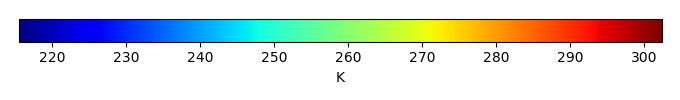MODEL MEANBIAS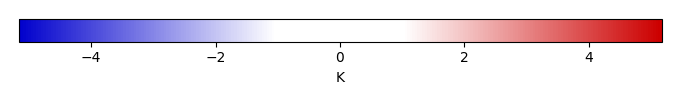BIAS SCORERMSE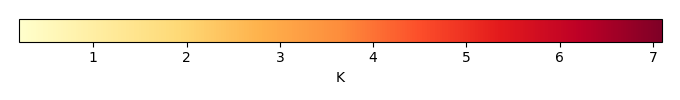RMSE SCOREBENCHMARK INTERANNUAL VARIABILITY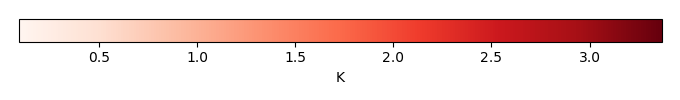MODEL INTERANNUAL VARIABILITYINTERANNUAL VARIABILITY SCOREBENCHMARK MAX MONTHMODEL MAX MONTHDIFFERENCE IN MAX MONTH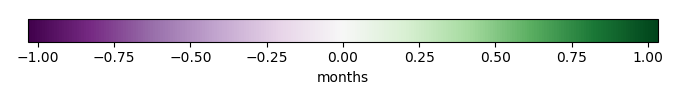SEASONAL CYCLE SCORESPATIAL TAYLOR DIAGRAMMODEL COLORS# Spatially integrated regional mean

MODEL COLORSREGIONAL MEANANNUAL CYCLEMONTHLY ANOMALYANNUAL CYCLE# All Models

BenchmarkCRUNCEPv7GSWP3v1WFDEI# Data Information

creation_date: Fri Nov 1 11:52:30 PDT 2013

source_file: This product is generated from monthly CRU TS3.21 Mean Temperature observations

title: derived CRU high resolution (0.5x0.5) Mean Temperature

Approach: I simply read the monthly surface air temperature from original file, and separated the time series by a month and saved them by each month and each year.

Temporal resolution: monthly

General information: This product was derived from CRU v3.21 product.

Spatial resolution: 0.5x0.5 degree